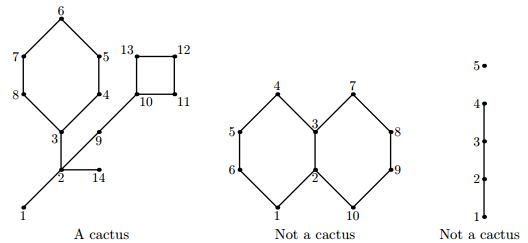Competitions

# Cactus

Cactus is a connected undirected graph in which every edge lies on at most one simple cycle. Intuitively cactus is a generalization of a tree where some cycles are allowed. Your task first is to verify if the given graph is a cactus or not. Important difference between a cactus and a tree is that a cactus can have a number of spanning subgraphs that are also cactuses. The number of such subgraphs (including the graph itself) determines cactusness of a graph (this number is one for a cactus that is just a tree). The cactusness of a graph that is not a cactus is considered to be zero.The first graph on the picture is a cactus with cactusness 35. The second graph is not a cactus because edge (2, 3) lies on two cycles. The third graph is not a cactus because it is not connected.

#### Input

The first line contains two integer numbers n and m (1n20000, 0m1000). Here n is the number of vertices in the graph. Vertices are numbered from 1 to n. Edges of the graph are represented by a set of edge-distinct paths, where m is the number of such paths.

Each of the following m lines contains a path in the graph. A path starts with an integer number ki (2ki1000) followed by ki integers from 1 to n. These ki integers represent vertices of a path. Path can go to the same vertex multiple times, but every edge is traversed exactly once in the whole input data. There are no multiedges in the graph (there is at most one edge between any two vertices).

#### Output

Write a single integer number - the cactusness of the given graph. Note that cactusness can be quite a large number.

Time limit 1 second
Memory limit 64 MiB
Input example #1
14 3
9 1 2 3 4 5 6 7 8 3
7 2 9 10 11 12 13 10
2 2 14

Output example #1
35

Source 2005 ACM NEERC , Semifinals, November 30, Problem C